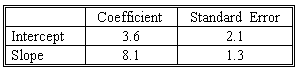### CFA Practice Question

There are 208 practice questions for this study session.

### CFA Practice Question

The results of the regressions using 200 observations on a variable Y against X are as follows:R square = 49%

The regression equation can be expressed as:
A. Y = 8.1 + 3.6 X + error
B. X = 8.1 + 3.6 Y + error
C. Y = 3.6 + 8.1 X + error
Explanation: Note that Y is regressed against X, implying that Y is the dependent (left-hand side) variable and X is the independent (right-hand side) variable. The intercept in a regression equals the constant term and the slope coefficient is the multiplier on the independent variable.